## Prime-Generating Polynomial

Legendreshowed that there is no Rational algebraic function which always gives Primes. In 1752, Goldbach showed that no Polynomial with Integer Coefficients can give a Prime for all integral values. However, there exists a Polynomial in 10 variables with Integer Coefficients such that the set of Primes equals the set of Positive values of this Polynomial obtained as the variables run through all Nonnegative Integers, although it is really a set of Diophantine Equations in disguise (Ribenboim 1991).Range Consecutive Reference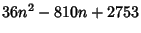[0, 44] 45 Fung and Ruby (Mollin and Williams 1990)[0, 42] 43 Fung and Ruby (Mollin and Williams 1990)[0, 39] 40 Euler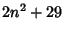[0, 28] 29 Legendre[0, 15] 16 Legendre[0, 10] 11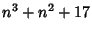[0, 10] 11

The above table gives some low-order polynomials which generate only Primes for the first few Nonnegative values (Mollin and Williams 1990). The best-known of these formulas is that due to Euler (Euler 1772, Ball and Coxeter 1987). Le Lionnais (1983) has christened numberssuch that the Euler-like polynomial(1)

is Prime for, 1, ...,as Lucky Numbers of Euler (where the casecorresponds to Euler's formula). Rabinowitz (1913) showed that for a Prime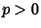, Euler's polynomial represents a Prime for(excluding the trivial case) Iff the Fieldhas Class Number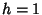(Rabinowitz 1913, Le Lionnais 1983, Conway and Guy 1996). As established by Stark (1967), there are only nine numbers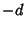such that(the Heegner Numbers,,,,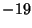,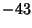,, and), and of these, only 7, 11, 19, 43, 67, and 163 are of the required form. Therefore, the only Lucky Numbers of Euler are 2, 3, 5, 11, 17, and 41 (Le Lionnais 1983, Sloane's A014556), and there does not exist a better prime-generating polynomial of Euler'sform.

Euleralso considered quadratics of the form(2)

and showed this gives Primes for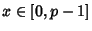for PrimeIffhas Class Number 2, which permits only, 5, 11, and 29. Baker (1971) and Stark (1971) showed that there are no such Fields for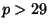. Similar results have been found for Polynomials of the form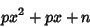(3)

(Hendy 1974).

See also Class Number, Heegner Number, Lucky Number of Euler, Prime Arithmetic Progression, Prime Diophantine Equations, Schinzel's Hypothesis

References

Abel, U. and Siebert, H. Sequences with Large Numbers of Prime Values.'' Am. Math. Monthly 100, 167-169, 1993.

Baker, A. Linear Forms in the Logarithms of Algebraic Numbers.'' Mathematika 13, 204-216, 1966.

Baker, A. Imaginary Quadratic Fields with Class Number Two.'' Ann. Math. 94, 139-152, 1971.

Ball, W. W. R. and Coxeter, H. S. M. Mathematical Recreations and Essays, 13th ed. New York: Dover, p. 60, 1987.

Boston, N. and Greenwood, M. L. Quadratics Representing Primes.'' Amer. Math. Monthly 102, 595-599, 1995.

Conway, J. H. and Guy, R. K. The Nine Magic Discriminants.'' In The Book of Numbers. New York: Springer-Verlag, pp. 224-226, 1996.

Courant, R. and Robbins, H. What is Mathematics?: An Elementary Approach to Ideas and Methods, 2nd ed. Oxford, England: Oxford University Press, p. 26, 1996.

Euler, L. Nouveaux Mémoires de l'Académie royale des Sciences. Berlin, p. 36, 1772.

Forman, R. Sequences with Many Primes.'' Amer. Math. Monthly 99, 548-557, 1992.

Garrison, B. Polynomials with Large Numbers of Prime Values.'' Amer. Math. Monthly 97, 316-317, 1990.

Hendy, M. D. Prime Quadratics Associated with Complex Quadratic Fields of Class Number 2.'' Proc. Amer. Math. Soc. 43, 253-260, 1974.

Le Lionnais, F. Les nombres remarquables. Paris: Hermann, pp. 88 and 144, 1983.

Mollin, R. A. and Williams, H. C. Class Number Problems for Real Quadratic Fields.'' Number Theory and Cryptology; LMS Lecture Notes Series 154, 1990.

Rabinowitz, G. Eindeutigkeit der Zerlegung in Primzahlfaktoren in quadratischen Zahlkörpern.'' Proc. Fifth Internat. Congress Math. (Cambridge) 1, 418-421, 1913.

Ribenboim, P. The Little Book of Big Primes. New York: Springer-Verlag, 1991.

Sloane, N. J. A. Sequence A014556 in The On-Line Version of the Encyclopedia of Integer Sequences.'' http://www.research.att.com/~njas/sequences/eisonline.html.

Stark, H. M. A Complete Determination of the Complex Quadratic Fields of Class Number One.'' Michigan Math. J. 14, 1-27, 1967.

Stark, H. M. An Explanation of Some Exotic Continued Fractions Found by Brillhart.'' In Computers in Number Theory, Proc. Science Research Council Atlas Symposium No. 2 held at Oxford, from 18-23 August, 1969 (Ed. A. O. L. Atkin and B. J. Birch). London: Academic Press, 1971.

Stark, H. M. A Transcendence Theorem for Class Number Problems.'' Ann. Math. 94, 153-173, 1971.